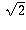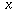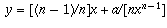Navigation Panel:(These buttons explained below)Question Corner and Discussion Area

# Calculating Square Roots

Asked by A. Stone and Sonya Baker on January 13, 1997:
What is the formula for finding square roots?

Thanks

The simplest, but most tedious, way to calculate the decimal digits of a square root is to figure out each digit in turn, as follows.

First of all, find how many digits to the left of the decimal point your square root will have.

A number with one digit to the left of the decimal point will be somewhere from 1 up to, but not including, 10; therefore, its square will be somewhere from 1 up to, but not including, 100, and hence will have either one or two digits to the left of the decimal point.

A number with two digits to the left of the decimal point will be somewhere from 10 up to, but not including, 100; therefore, its square will be somewhere from 100 up to, but not including, 10000, and hence will have either three or four digits to the left of the decimal point.

And so on.

Therefore, if the number you're trying to find the square root of has one or two digits to the left of the decimal point, its square root will have one; if it has three or four, its square root will have two; and so on.

Now, having found how many digits the square root has, just start from the left and figure out each digit in turn.

For example, to find the square root of 1234 to 3 decimal places:

The square root has two digits to the left of the decimal point, so we're looking for a number of the form AB.CDE. . . (and we want to find A through E).

Now, 10^2=100, 20^2 = 400, 30^2=900, all too small, but 40^2=1600, too big. This tells us the first digit A is 3.

Next, 31^2 = 961, 32^2 = 1024, 33^2 = 1089, 34^2=1156, 35^2 = 1225, all too small; 36^2 = 1296, too big, so the second digit B is 5.

Thirdly, 35.1^2 = 1232.01, too small, but 35.2^2 = 1239.04, too big, so the third digit C is 1.

And so on; continuing in this way, you see that.

This method is horribly slow and inefficient but that's no problem in an era of calculators. All the necessary ideas are there.

You can apply lots of little shortcuts to this procedure to drastically reduce the number of computations you need to do. Then you come up with a procedure similar to the long-division algorithm. Its complicated to remember, though; I don't remember it offhand. It is seldom taught today because there is little need for it.

To actually calculate a square root to a large number of digits quickly, one uses a different method. For example, saying that x^2 = a is the same as saying x = (1/2)(x + a/x) and you can use this as the basis of an iteration. Start with one value of x, for example, x=1. Then get a second value y = (1/2)(x + a/x). Then get a third value z = (1/2)(y + a/y). And so on. It turns out that these numbers x, y, z, . . . converge quite quickly to the desired square root, especially if you start with a number that is already close.

Doing this calculation in the above example, for a=1234, and starting with the number a=40 which is obviously just a little bit bigger than the desired square root, you get the numbers 40, 35.425, 35.129578. . . , 35.128336. . . , 35.128336. . . and you see that just four calculations are enough to get the square root accurate to six decimal places.

What is the square root of 2?
The square root of two is the one and only positive real number whose square is 2. That's really the only way to answer the question "what is the square root of 2".

Now, it's possible you may have had a different question in mind: what are the digits of the square root of 2?

The digits of an irrational number like the square root of 2 (a number which is not a ratio of two integers) do not ever reach a repeating pattern the way digits of rational numbers do. Therefore, you cannot simply write down all the digits of an irrational number, for there are infinitely many of them and they don't repeat. However, you can write down as many as you like, using methods such as that described in the question above. The first few digits of the square root of 2 are 1.41421356. . . .

Because writing down digits like that doesn't completely and unambiguously identify the number (for instance, I wrote the first 8 digits after the decimal point, but that doesn't tell you what the ninth is), a mathematician would never say that "1.41421356. . . " is an "answer" to the question "what is the square root of 2". Rather, the correct answer to the question is the first answer I gave above: "the one and only positive real number whose square is 2". That is what the square root of 2 actually is. Other responses, such as writing down digits, are useful in understanding the properties of, such as where it fits in to the number line and how it compares with other more familiar numbers, but they are not answers to the question of what it is.

Asked by a student at Alfred M. Barbe school on May 1, 1997:
What is the technical name for the square root symbol (radix)?
It can be referred to as either a "radical sign" or a "surd symbol" (or various other combinations of these, such as "radical symbol").

I believe it was originally derived from a corrupted form of the initial letter of the word "radix", so "radical sign" might be the most appropriate.

Asked by an anonymous poster on October 17, 1997:

I read the method of calculating square root I wanted to know if there is a way to calculate any degree of root 3,4 and so on what about rational roots 2.5 and so on is there any methods for those? I thank you for your time and if you would be able to send me an e-mail notice when the answer will be posted I will thank you more p.s. your site is the most informative and useful even for university student in math and science department as myself
The methods for computing square roots illustrated above can be generalized to handle any rational exponent. We will first consider the case in which the degree of the root to be taken is a whole number n. Let a be the number of which we wish to take the nth root. As before, first find the greatest power of 10 which is less than the nth root of a. Increase the leading digit of this power of 10 until you reach a number such that if you increase the leading digit one more time, the nth power is greater than a. Move to the next digit and repeat the process.

If a is 7809 and n is 3 then 10 is the greatest power of 10 whose 3rd power (1000) is less than 7809. Increasing the leading digit of 10, we find that 10 cubed is less than 7809 but 20 cubed is greater. This shows our desired root is of the form 1A.BCD. . . . Going to the next digit, we note that 19 cubed is 6859 but 20 cubed is 8000. This shows our desired root is of the form 19.BCD. . . . We continue the process as long as is necessary to obtain the desired number of digits of the root.

If the degree of the root is a fraction, i.e. we are trying to computefor some integers m and n, then we first take the nth root of a and then raise this number to the mth power. Note that the degree of the root is the reciprocal of the exponent of a:.

There is a faster approach which can be used, though the reason why it works is not quite so simple. We first need a rough estimateof the value of root. From this we compute. Now if our first guess was reasonably close to the actual value of the root, then y will be even closer. If we then repeat the process using y for our guess, the new valuewhich we get will be closer still. The sequence of numbers x, y, z, . . . obtained in this way converges quite quickly to the desired root, as long as the initial guess was close enough.

[ Submit Your Own Question ] [ Create a Discussion Topic ]

This part of the site maintained by (No Current Maintainers)
Last updated: April 19, 1999
Original Web Site Creator / Mathematical Content Developer: Philip Spencer
Current Network Coordinator and Contact Person: Joel Chan - mathnet@math.toronto.edu

Navigation Panel: